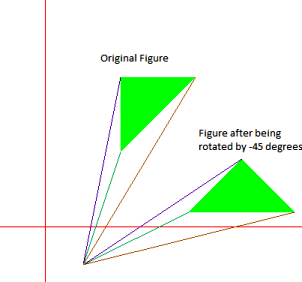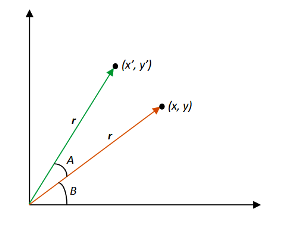GeeksforGeeks App
Open AppBrowser
Continue

# 2D Transformation | Rotation of objects

We have to rotate an object by a given angle about a given pivot point and print the new co-ordinates.
Examples:

Input : {(100, 100), (150, 200), (200, 200),
(200, 150)} is to be rotated about
(0, 0) by 90 degrees
Output : (-100, 100), (-200, 150), (-200, 200), (-150, 200)Input : {(100, 100), (100, 200), (200, 200)}
is to be rotated about (50, -50) by
-45 degrees
Output : (191.421, 20.7107), (262.132, 91.4214),
(332.843, 20.7107)In order to rotate an object we need to rotate each vertex of the figure individually.
On rotating a point P(x, y) by an angle A about the origin we get a point P'(x’, y’). The values of x’ and y’ can be calculated as follows:-We know that,
x = rcosB, y = rsinB
x’ = rcos(A+B) = r(cosAcosB – sinAsinB) = rcosBcosA – rsinBsinA = xcosA – ysinA
y’ = rsin(A+B) = r(sinAcosB + cosAsinB) = rcosBsinA + rsinBcosA = xsinA + ycosA
Rotational Matrix Equation:-## CPP

 // C++ program to rotate an object by// a given angle about a given point#include #include using namespace std; // Using macros to convert degree to radian// and call sin() and cos() as these functions// take input in radians#define SIN(x) sin(x * 3.141592653589 / 180)#define COS(x) cos(x * 3.141592653589 / 180) // To rotate an object given as order set of points in a[]// (x_pivot, y_pivot)void rotate(float a[], int n, int x_pivot, int y_pivot,            int angle){    int i = 0;    while (i < n) {        // Shifting the pivot point to the origin        // and the given points accordingly        int x_shifted = a[i] - x_pivot;        int y_shifted = a[i] - y_pivot;         // Calculating the rotated point co-ordinates        // and shifting it back        a[i] = x_pivot                  + (x_shifted * COS(angle)                     - y_shifted * SIN(angle));        a[i] = y_pivot                  + (x_shifted * SIN(angle)                     + y_shifted * COS(angle));        cout << "(" << a[i] << ", " << a[i] << ") ";        i++;    }} // Driver Codeint main(){    // 1st Example    // The following figure is to be    // rotated about (0, 0) by 90 degrees    int size1 = 4; // No. of vertices    // Vertex co-ordinates must be in order    float points_list1[] = { { 100, 100 },                                { 150, 200 },                                { 200, 200 },                                { 200, 150 } };    rotate(points_list1, size1, 0, 0, 90);     // 2nd Example    // The following figure is to be    // rotated about (50, -50) by -45 degrees    /*int size2 = 3;//No. of vertices    float points_list2[] = {{100, 100}, {100, 200},                                {200, 200}};    rotate(points_list2, size2, 50, -50, -45);*/    return 0;}

## Java

 // Java program to rotate an object by// a given angle about a given pointpublic class rotation {   static void rotate(double a[][], int n, int x_pivot,                     int y_pivot, int angle)  {    int i = 0;    while (i < n)    {             // Shifting the pivot point to the origin      // and the given points accordingly      int x_shifted = (int)a[i] - x_pivot;      int y_shifted = (int)a[i] - y_pivot;       // Calculating the rotated point co-ordinates      // and shifting it back      double x = Math.toRadians(angle);      a[i] = x_pivot        + (x_shifted * Math.cos(x)           - y_shifted * Math.sin(x));      a[i] = y_pivot        + (x_shifted * Math.sin(x)           + y_shifted * Math.cos(x));      System.out.printf("(%f, %f) ", a[i],                        a[i]);      i++;    }  }   // Driver Code  public static void main(String[] args)  {    // 1st Example    // The following figure is to be    // rotated about (0, 0) by 90 degrees    int size1 = 4; // No. of vertices     // Vertex co-ordinates must be in order    double points_list1[][] = { { 100, 100 },                               { 150, 200 },                               { 200, 200 },                               { 200, 150 } };    rotate(points_list1, size1, 0, 0, 90);     // 2nd Example    // The following figure is to be    // rotated about (50, -50) by -45 degrees    /*int size2 = 3;//No. of vertices        double points_list2[] = {{100, 100}, {100, 200},                                    {200, 200}};        rotate(points_list2, size2, 50, -50, -45);*/  }} // This code is contributed by karandeep1234

## Python3

 # Python3 program to rotate an object by# a given angle about a given pointimport math SIN=lambda x: int(math.sin(x * 3.141592653589 / 180))COS=lambda x: int(math.cos(x * 3.141592653589 / 180)) # To rotate an objectdef rotate(a, n, x_pivot, y_pivot, angle):    i = 0    while (i < n) :        # Shifting the pivot point to the origin        # and the given points accordingly        x_shifted = a[i] - x_pivot        y_shifted = a[i] - y_pivot         # Calculating the rotated point co-ordinates        # and shifting it back        a[i] = x_pivot + (x_shifted * COS(angle) - y_shifted * SIN(angle))        a[i] = y_pivot + (x_shifted * SIN(angle) + y_shifted * COS(angle))        print("({}, {}) ".format(a[i], a[i]),end=" ")        i+=1       # Driver Codeif __name__=='__main__':    # 1st Example    # The following figure is to be    # rotated about (0, 0) by 90 degrees    size1 = 4 # No. of vertices     # Vertex co-ordinates must be in order    points_list1 = [[ 100, 100],                    [ 150, 200],                    [ 200, 200],                    [ 200, 150],]     rotate(points_list1, size1, 0, 0, 90)     # 2nd Example    # The following figure is to be    # rotated about (50, -50) by -45 degrees    # size2 = 3#No. of vertices    # points_list2 = [[100, 100],    #                     [100, 200],    #                     [200, 200]]    # rotate(points_list2, size2, 50, -50, -45)

## Javascript

 // Javascript program to rotate an object by// a given angle about a given pointconst SIN = (x) => Math.sin(x * Math.PI / 180);const COS = (x) => Math.cos(x * Math.PI / 180); function rotate(a, n, x_pivot, y_pivot, angle) {  let i = 0;  while (i < n) {    // Shifting the pivot point to the origin    // and the given points accordingly    const x_shifted = a[i] - x_pivot;    const y_shifted = a[i] - y_pivot;     // Calculating the rotated point co-ordinates    // and shifting it back    a[i] = x_pivot + (x_shifted * COS(angle) - y_shifted * SIN(angle));    a[i] = y_pivot + (x_shifted * SIN(angle) + y_shifted * COS(angle));    console.log((${a[i]},${a[i]}) );    i++;  }} // Driver Code// 1st Example// The following figure is to be// rotated about (0, 0) by 90 degreesconst size1 = 4; // No. of vertices // Vertex co-ordinates must be in orderconst points_list1 = [[ 100, 100],                      [ 150, 200],                      [ 200, 200],                      [ 200, 150],];rotate(points_list1, size1, 0, 0, 90); // 2nd Example// The following figure is to be// rotated about (50, -50) by -45 degrees// const size2 = 3; // No. of vertices// const points_list2 = [[100, 100],//                       [100, 200],//                       [200, 200]];// rotate(points_list2, size2, 50, -50, -45);

## C#

 // C# Program to rotate an object by// a given angle about a given pointusing System;   class rotation{    // Function to rotate the given points    // about the pivot point by angle    static void rotate(double[,] a, int n,                       int x_pivot, int y_pivot, int angle)    {        int i = 0;        while (i < n)        {            // Shifting the pivot point to the origin            // and the given points accordingly            int x_shifted = (int)a[i, 0] - x_pivot;            int y_shifted = (int)a[i, 1] - y_pivot;               // Calculating the rotated point co-ordinates            // and shifting it back            double x = Math.PI * angle / 180.0;            a[i, 0] = x_pivot + (x_shifted *                                   Math.Cos(x) - y_shifted *                                   Math.Sin(x));            a[i, 1] = y_pivot + (x_shifted *                                   Math.Sin(x) + y_shifted *                                   Math.Cos(x));            Console.Write("({0}, {1}) ",                          a[i, 0], a[i, 1]);            i++;        }    }       // Driver Code    public static void Main(String[] args)    {        // 1st Example        // The following figure is to be        // rotated about (0, 0) by 90 degrees        int size1 = 4; // No. of vertices           // Vertex co-ordinates must be in order        double[,] points_list1 = { { 100, 100 },                               { 150, 200 },                               { 200, 200 },                               { 200, 150 } };        rotate(points_list1, size1, 0, 0, 90);           // 2nd Example        // The following figure is to be        // rotated about (50, -50) by -45 degrees        /*int size2 = 3;//No. of vertices        double[,] points_list2 = { { 100, 100 },                                  { 100, 200 },                                  { 200, 200 } };        rotate(points_list2, size2, 50, -50, -45);*/    }}

Output:

(-100, 100), (-200, 150), (-200, 200), (-150, 200)

Time Complexity: O(N)
Auxiliary Space: O(1)
References: Rotation matrix

This article is contributed by Nabaneet Roy. If you like GeeksforGeeks and would like to contribute, you can also write an article using write.geeksforgeeks.org or mail your article to review-team@geeksforgeeks.org. See your article appearing on the GeeksforGeeks main page and help other Geeks.